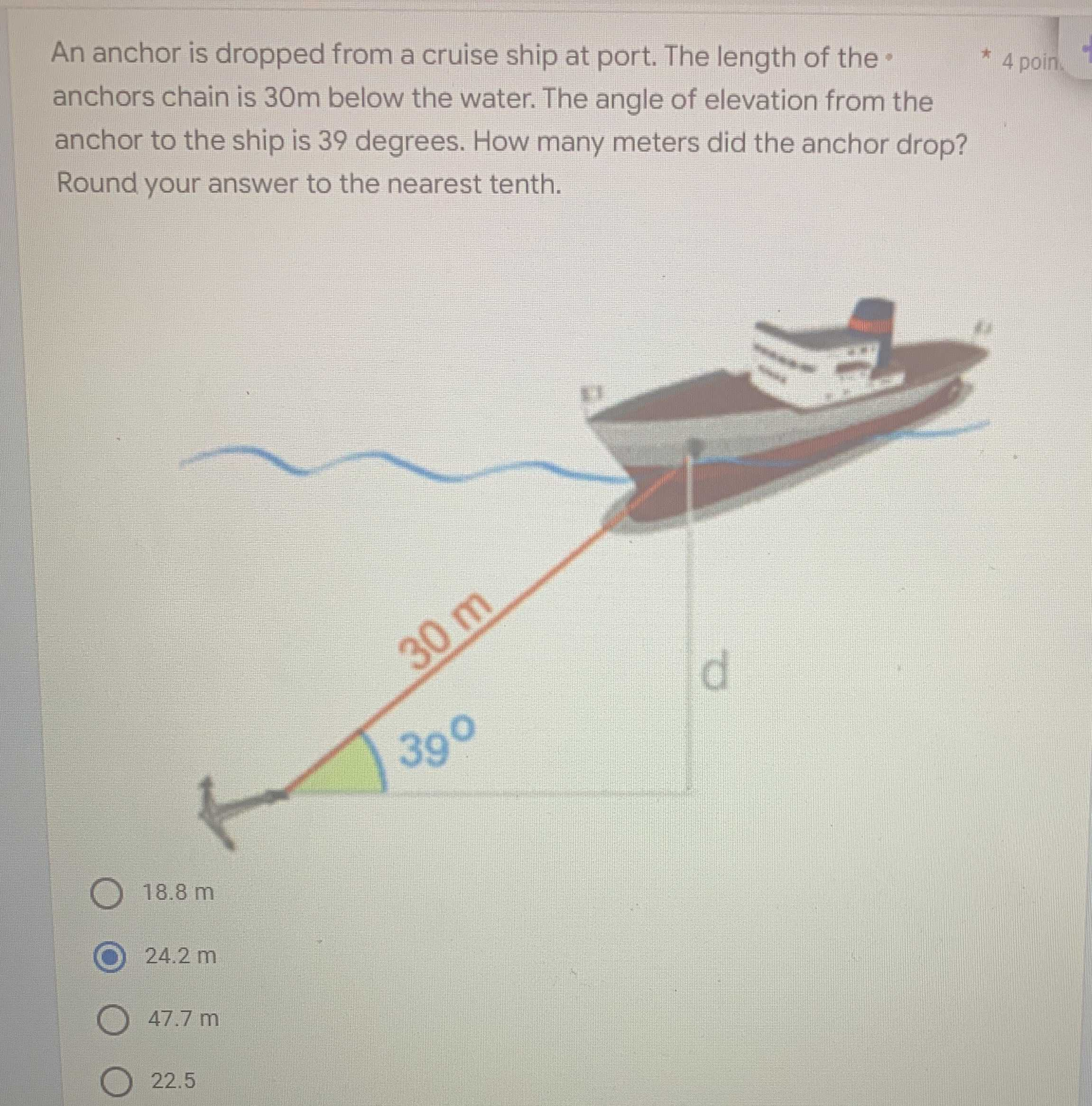### Still have math questions?

Trigonometry
QuestionAn anchor is dropped from a cruise ship at port. The length of the  anchors chain is $$30 m$$ below the water. The angle of elevation from the anchor to the ship is $$39$$ degrees. How many meters did the anchor drop? Round your answer to the nearest tenth.

$$18.8 m$$

$$24.2 m$$

$$47.7 m$$

$$22.5$$

$$d= 30\sin39= 18.8$$